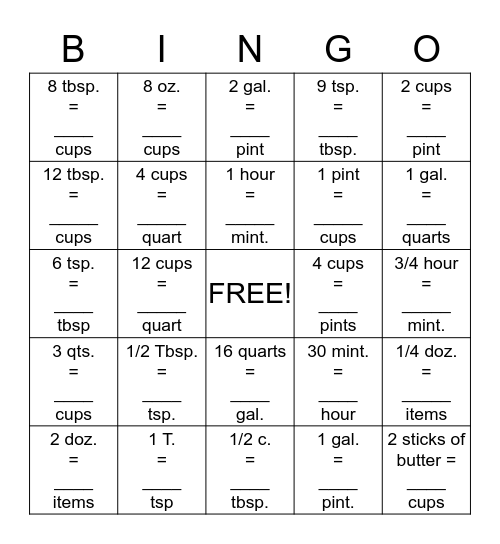# Equivalents BingoThis bingo card has a free space and 24 words: 1/2 Tbsp. = ____ tsp., 1 T. = ____ tsp, 1 hour = _____ mint., 2 doz. = ____ items, 2 cups = ____ pint, 8 tbsp. = ____ cups, 12 tbsp. = _____ cups, 8 oz. = ____ cups, 4 cups = ____ pints, 16 quarts = ____ gal., 1 pint = _____ cups, 6 tsp. = ____ tbsp, 30 mint. = ____ hour, 1/4 doz. = _____ items, 4 cups = _____ quart, 1 gal. = ____ pint., 3/4 hour = _____ mint., 2 gal. = ____ pint, 1 gal. = ____ quarts, 12 cups = _____ quart, 2 sticks of butter = ____ cups, 3 qts. = ____ cups, 9 tsp. = ____ tbsp. and 1/2 c. = ____ tbsp..

## Play Online